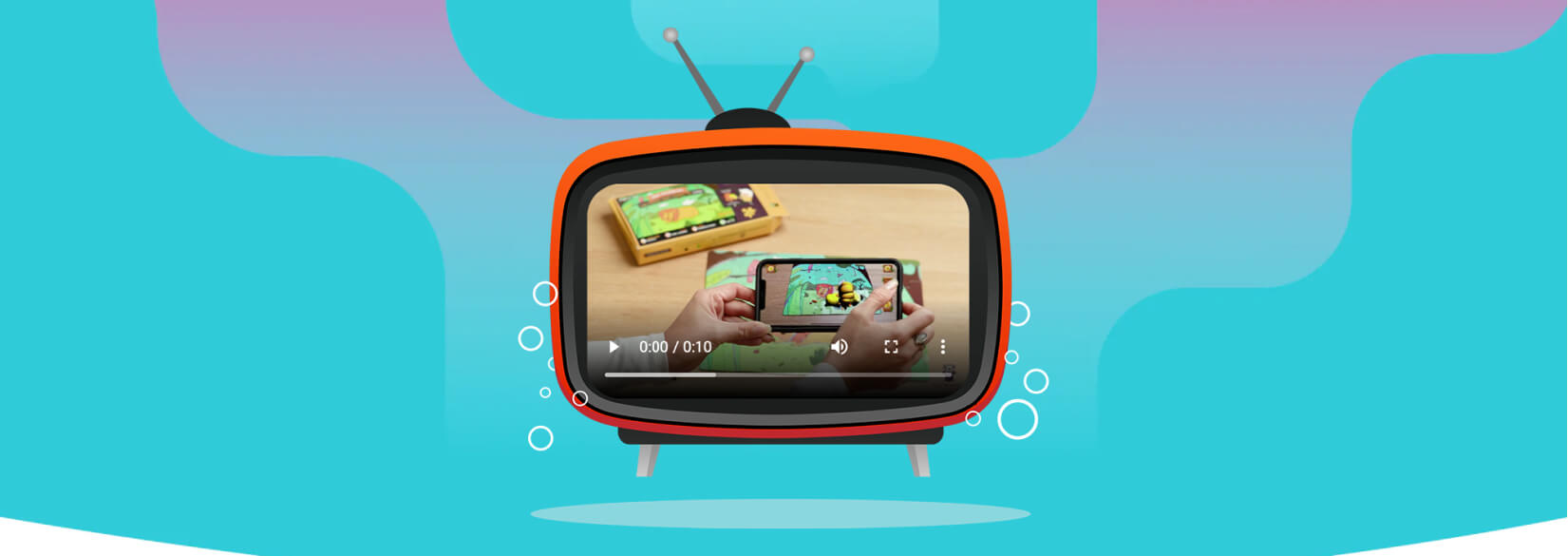# Case Study

## The Wunder CompanyThe Wunder Company combines physical toys and the magic of augmented reality to create an immersive learning experience for kids.## Problem

Here is where you add the problems. Here is where you add the problems. Here is where you add the problems. Here is where you add the problems. Here is where you add the problems. Here is where you add the problems. Here is where you add the problems.## Solution

Here is where you add the problems. Here is where you add the problems. Here is where you add the problems. Here is where you add the problems. Here is where you add the problems. Here is where you add the problems. Here is where you add the problems.## Outcome

Here is where you add the problems. Here is where you add the problems. Here is where you add the problems. Here is where you add the problems. Here is where you add the problems. Here is where you add the problems. Here is where you add the problems.## Website Design and Development

You can add the contents for this project here.